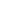# Ancient Mathematics from Babylon to Greece

\$75 Limited inc GST / \$68
Ancient Mathematics from Babylon to Greece

<p>Greeks inherited mathematical knowledge from ancient Egyptian and Babylonians, but they asked questions and searched for proof. From 6th century BCE to 4 century CE, starting from Thales of Miletus

...
Enrol into Ancient Egypt and Babylonian mathematics; Ancient Greek mathematics; and Geometry Along the Way and only pay \$150!
Mo Tu We Th Fr Sa SuIf there isn't a class to suit you, please the waiting list.

Greeks inherited mathematical knowledge from ancient Egyptian and Babylonians, but they asked questions and searched for proof. From 6th century BCE to 4 century CE, starting from Thales of Miletus and Pythagoras of Samos, to Aristotle and Euclid, and finishing with Diophantus and the only known female ancient Greek mathematician Hypatia, Greeks developed mathematics into deductive systematic science, with definitions, postulates, theorems, and proofs. They developed the theory of numbers, found all regular polyhedra, measured diameter of the Earth, proposed geocentric and heliocentric systems, and solved numerous theoretical and practical problems that became the basics of mathematics.

SUGGESTED READING
Science Awakening I: Egyptian, Babylonian and Greek Mathematics, B. L. Van Der Waerden. 2005, 4th Edition.

COURSE OUTLINE

• Main chronology and persons of ancient Greek mathematics.
• Greek numeral systems.
• Zeno’s paradoxes.
• Three great mathematical problems: doubling the cube; trisecting the angle; squaring the circle.
• Pythagorean number theory. Irrationality.
• Elements by Euclid.
• Archimedes: his mathematical discoveries, legends about him. Hypatia, a female mathematician.

PLANNED LEARNING OUTCOMES
By the end of this course, students should be able to:

1. Understand ancient Greek numeral systems.
2. Know main timeline of ancient Greek mathematics.
3. Know concepts of Pythagorean theory of harmony and theory of numbers.
4. Know Platonic regular polyhedra.
5. Become acquainted with axiomatic geometry by Euclid and Archimedes as a geometer and engineer.